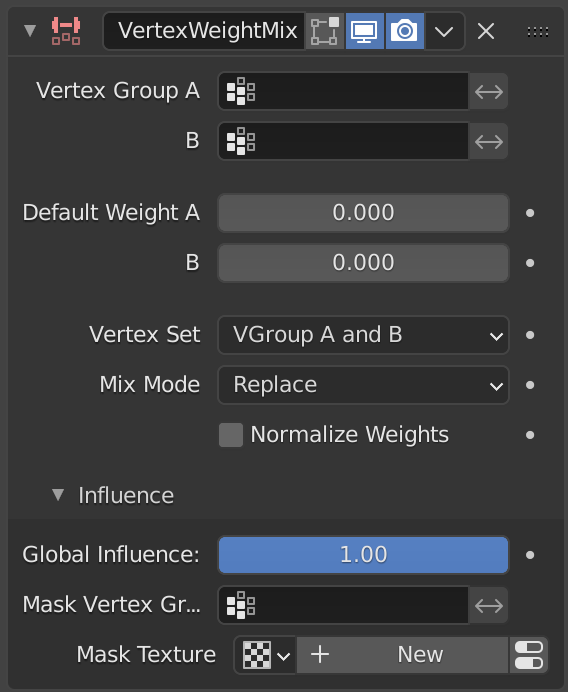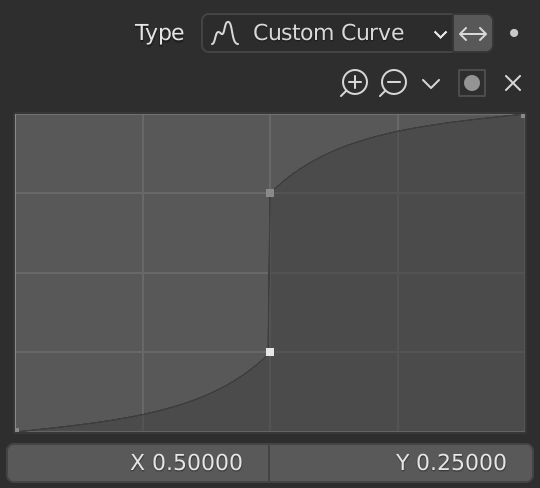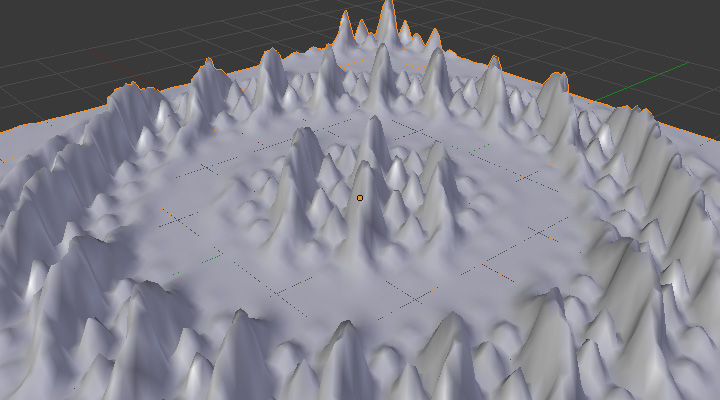# Vertex Weight Mix Modifier¶

This modifier mixes a second vertex group (or a simple value) into the affected vertex group, using different operations.

Wichtig

This modifier does implicit clamping of weight values in the standard (0.0 to 1.0) range. All values below 0.0 will be set to 0.0, and all values above 1.0 will be set to 1.0.

Bemerkung

You can view the modified weights in Weight Paint Mode. This also implies that you will have to disable the Vertex Weight Mix modifier if you want to see the original weights of the vertex group you are editing.

## Options¶The Vertex Weight Mix modifier panel.

Vertex Group A

The vertex group to affect.

Default Weight A

The default weight to assign to all vertices not in the given vertex group.

Vertex Group B

The second vertex group to mix into the affected one. Leave it empty if you only want to mix in a simple value.

Default Weight B

The default weight to assign to all vertices not in the given second vertex group.

Mix Mode

How the vertex group weights are affected by the other vertex group’s weights.

Replace weights

Replaces affected weights with the second group’s weights.

Adds the values of Group B to Group A.

Subtract from weights

Subtracts the values of Group B from Group A.

Multiply weights

Multiplies the values of Group B with Group A.

Divide weights

Divides the values of Group A by Group B.

Difference

Subtracts the smaller of the two values from the larger.

Average

Adds the values together, then divides by 2.

Mix Set

Choose which vertices will be affected.

All vertices

Affects all vertices, disregarding the vertex groups content.

Vertices from group A

Affects only vertices belonging to the affected vertex group.

Vertices from group B

Affects only vertices belonging to the second vertex group.

Vertices from one group

Affects only vertices belonging to at least one of the vertex groups.

Vertices from both groups

Affects only vertices belonging to both vertex groups.

Wichtig

When using All vertices, Vertices from group B or Vertices from one group, vertices might be added to the affected vertex group.A customized mapping curve.¶Custom Mapping disabled.¶